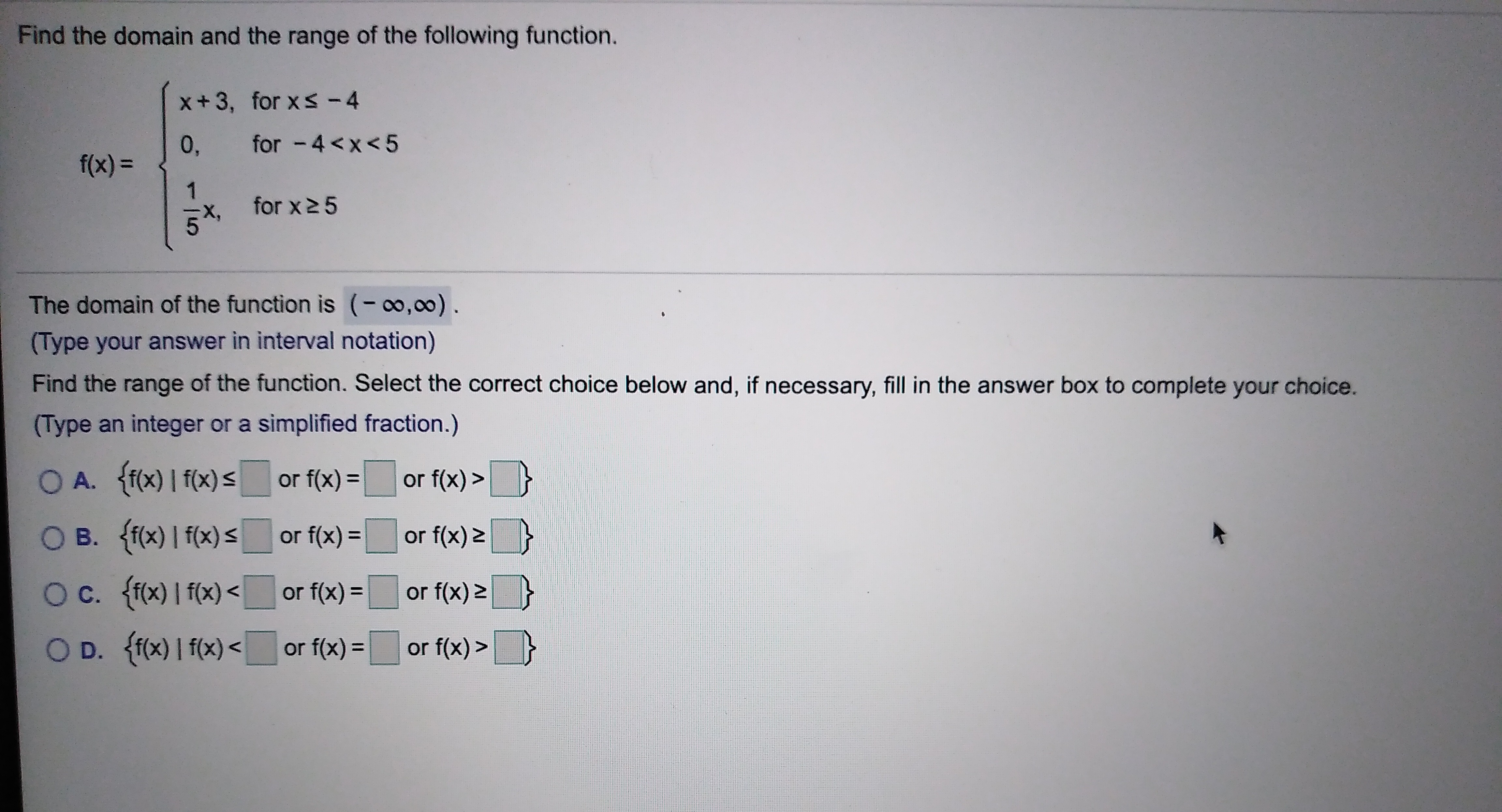# Find the domain and the range of the following function.x+ 3, for x < - 4for -4or f(x) =O B. ff(x) I f(x) sor f(x)=or f(x) 2O c. ffx) I f(x)or f(x) 2or f(x) =O D. ff0x) I f(x)f(x)=or f(x)>or

Question
5 viewshelp_outlineImage TranscriptioncloseFind the domain and the range of the following function. x+ 3, for x < - 4 for -4 or f(x) = O B. ff(x) I f(x) s or f(x)= or f(x) 2 O c. ffx) I f(x) or f(x) 2 or f(x) = O D. ff0x) I f(x) f(x)= or f(x)> or fullscreen
check_circle

Step 1

We are given the ...

### Want to see the full answer?

See Solution

#### Want to see this answer and more?

Solutions are written by subject experts who are available 24/7. Questions are typically answered within 1 hour.*

See Solution
*Response times may vary by subject and question.
Tagged in

### Equations and In-equations